Function Repository Resource:

# FrobeniusDSolve

Compute an approximate Frobenius or power series solution to an ODE

Contributed by: Rauan Kaldybayev
 ResourceFunction["FrobeniusDSolve"][eqn,u,x,x0,n] solves a differential equation for the function u in the independent variable x using a Frobenius or power series. ResourceFunction["FrobeniusDSolve"][x,x0,n,formula] uses the algebraic formula supplied by the resource function FrobeniusDSolveFormula to quickly compute the solution.

## Details

A Frobenius series has the form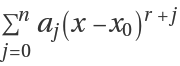.
The output is of the form {soln1,soln2,,solnZ}, where Z is the order of the ODE and soln1,soln2,,solnZ are linearly independent solutions to the ODE. They are of the form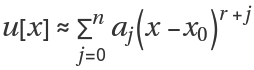, normalized by a0=1.
ResourceFunction["FrobeniusDSolve"] can solve linear homogeneous ODEs with polynomial coefficients.
Any linear ODE with polynomial coefficients can be written in the form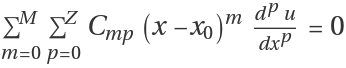for some (any) x0. Frobenius's method assumes solution of the form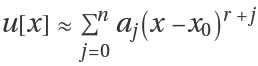; substituting this into the equation yields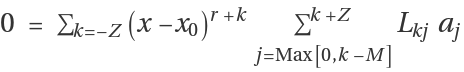, where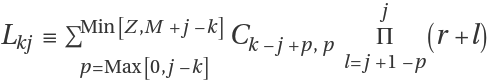. To satisfy the equation, the coefficients before all power of x-x0 must be zero: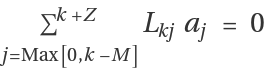for -Zk. From here, we can determine the possible values of the constant r for which a00, and then for each r, compute the coefficients. Read more at the related Wolfram Community post.
ResourceFunction["FrobeniusDSolve"] allows the option "ComputeError":
 "ComputeError" True compute the error of the approximate solution
If "ComputeError" is True, the output is of the form {{soln1,err1},{soln2,err2},{solnZ,errZ}}, where Z is the order of the ODE, {solnk} are the linearly independent (approximate) solutions to the ODE, and {errk} are the errors of the appoximate solutions {solnk}. The error of an approximate solution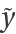to an ODE of the form Ly=0 is defined as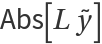. In other words, it is the absolute value of the remainder. The formulas for {errk} returned by ResourceFunction["FrobeniusDSolve"] are exact.
Approximate numbers make the function much faster while not affecting accuracy in a significant way. To solve a differential equation exactly using Frobenius's method, in general we would have to compute an infinite number of terms, which is of course impossible. Solutions obtained using Frobenius's method will thus always be approximate, even if exact numbers are used. Because the decrease in accuracy that comes from using approximate numbers is very small, using approximate numbers doesn't really affect the accuracy. And since doing computations with approximate numbers is much faster, they are used in ResourceFunction["FrobeniusDSolve"].

## Examples

### Basic Examples (2)

Obtain an order six approximate solution to the ordinary differential equation 2x2y''+9x(x+1)y'-3y=0 using a Frobenius series around the singular point x0=0:

 In:=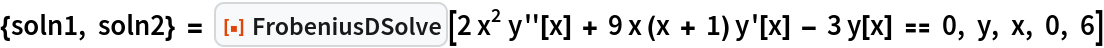Out=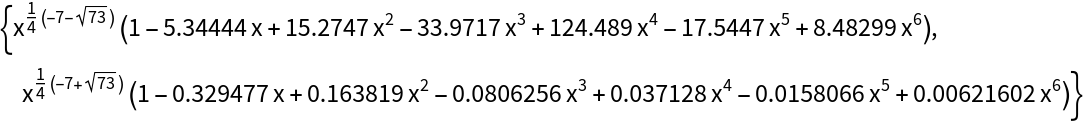Plot the solutions:

 In:=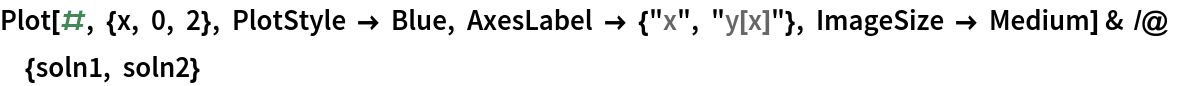Out=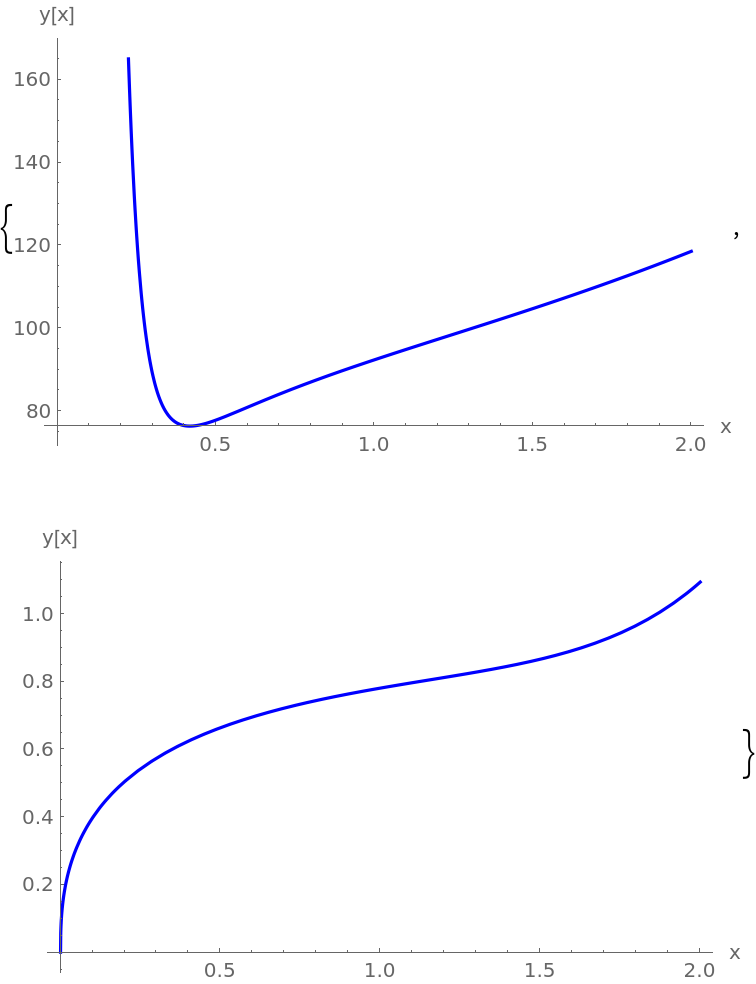Compute a more accurate solution with n=40 and compare to the previous result:

 In:=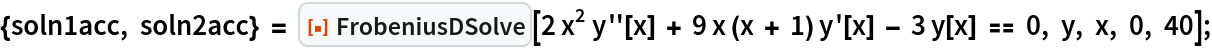In:=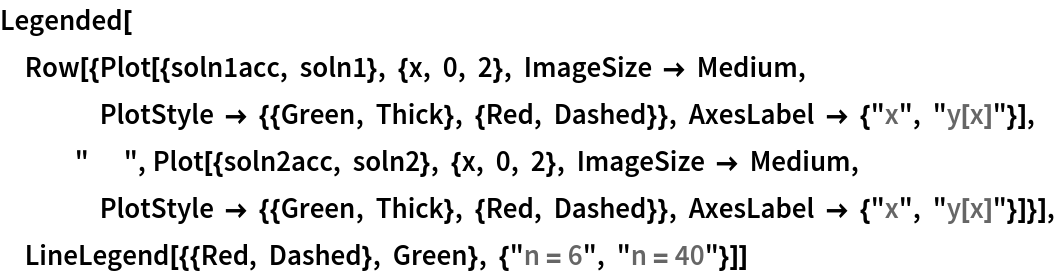Out=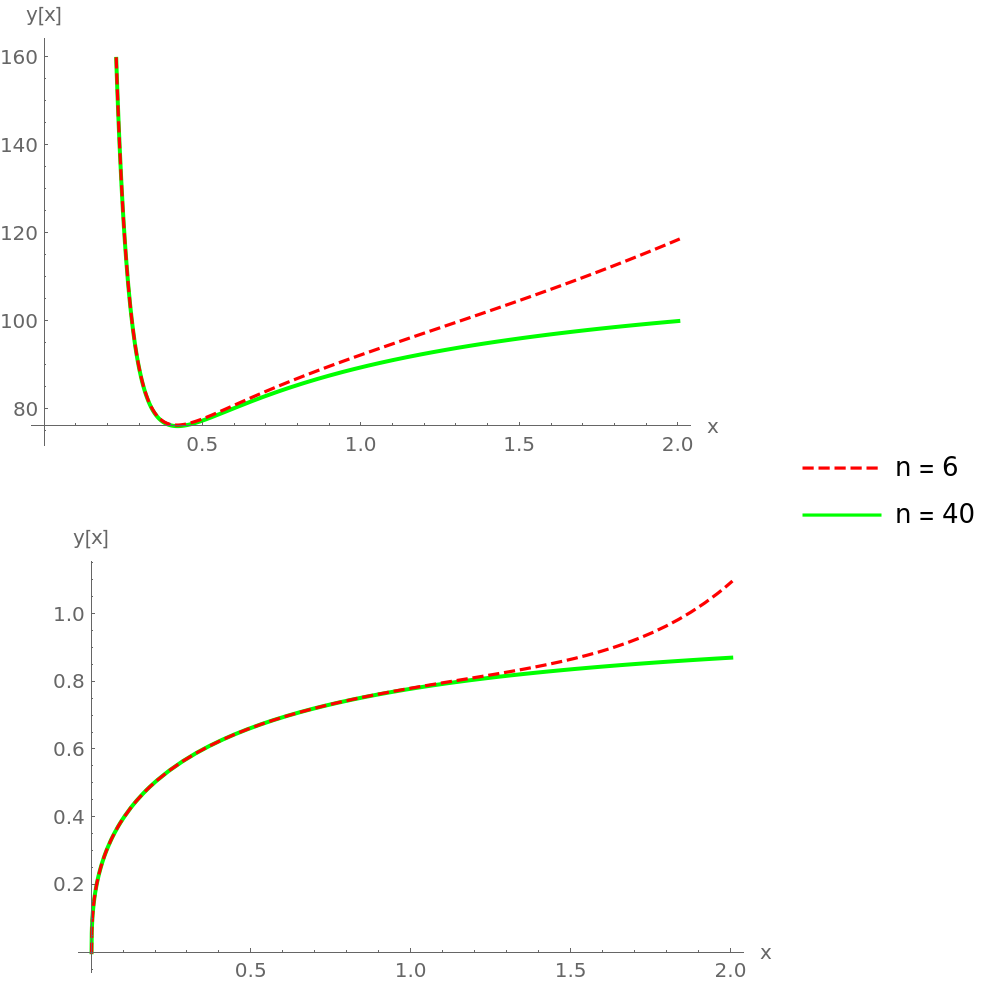Plot the residuals Abs[x2y''+9x(x+1)y'-3y]:

 In:=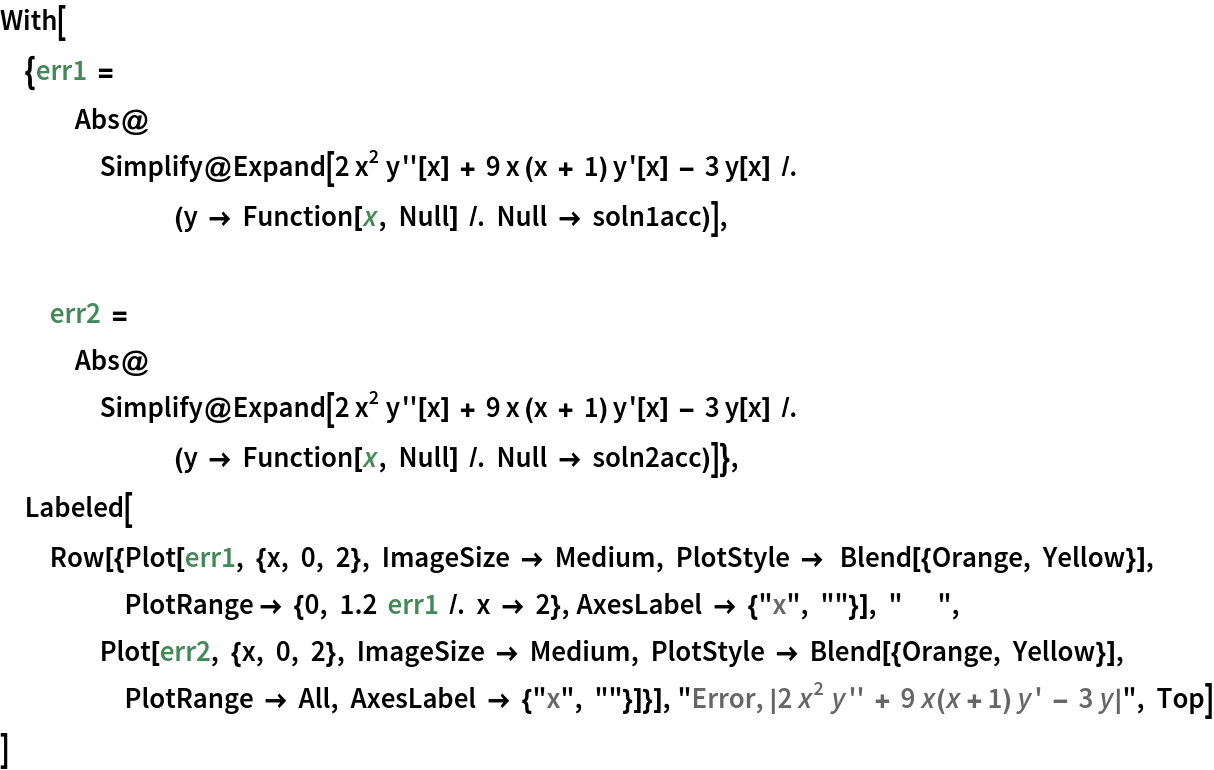Out=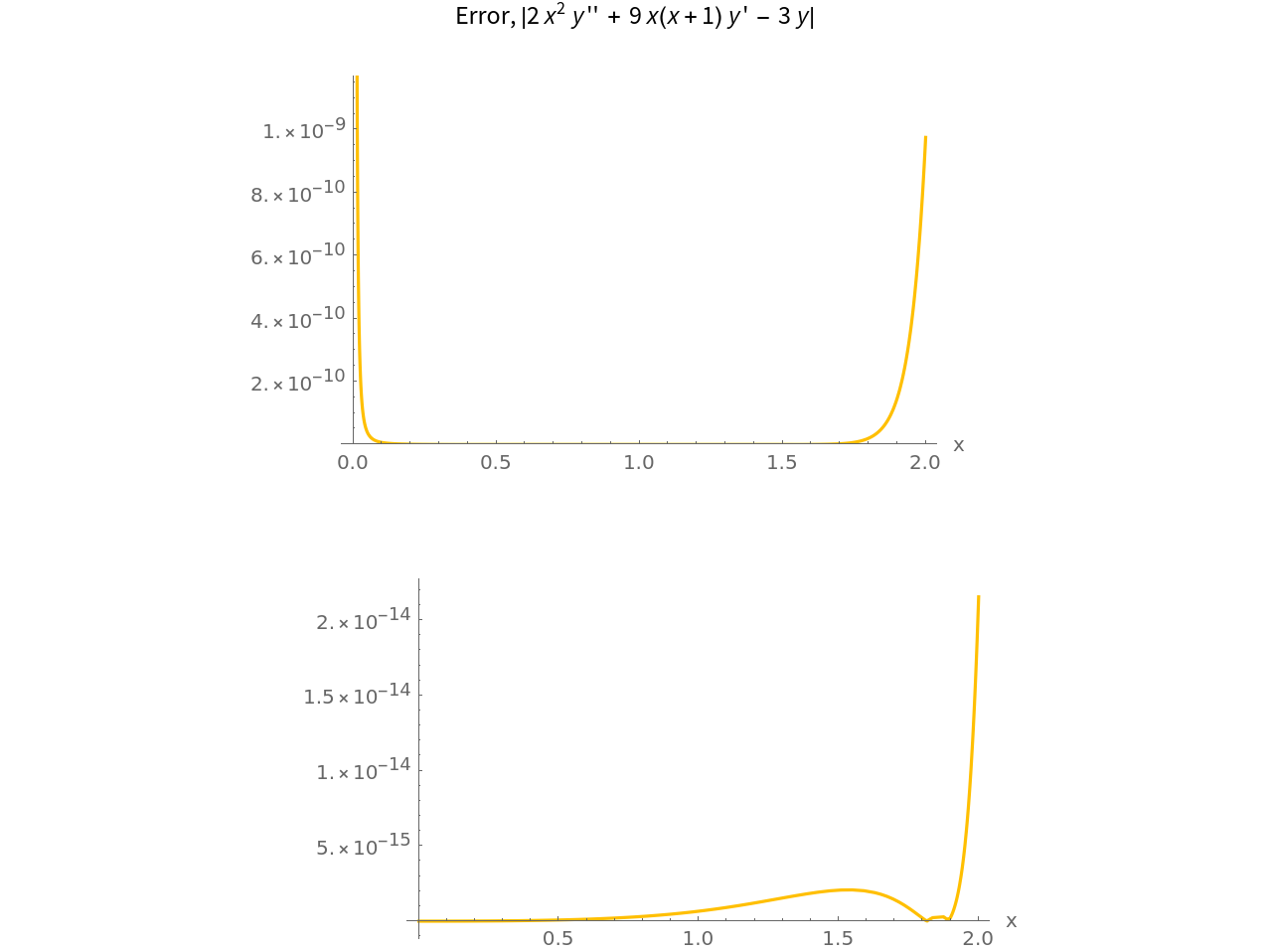As you can see, the solutions are very accurate. (see Author Notes for more information)

FrobeniusDSolve can also be used to solve equations that don't have singular points. Solve the pendulum equation θ''[t]=-θ[t] near the point t=-3, with n=16:

 In:=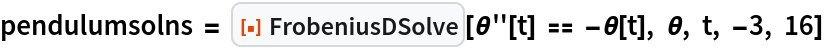Out=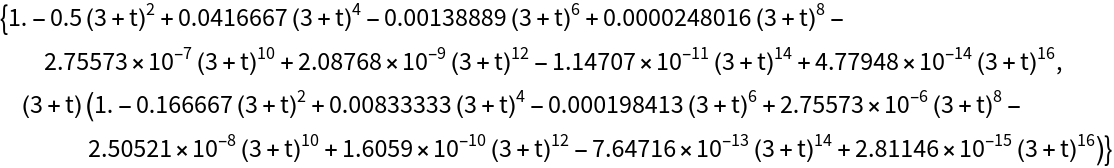Plot the result:

 In:=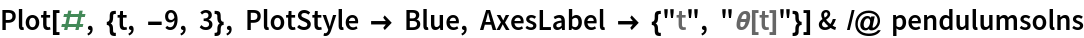Out=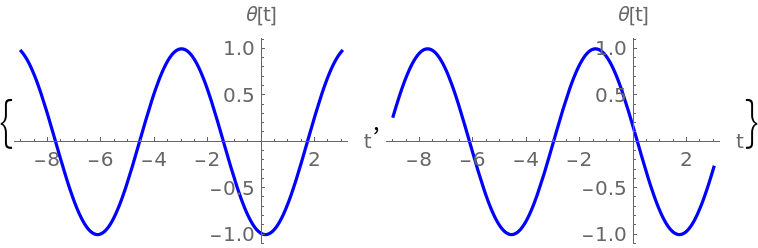### Scope (3)

The function can operate with complex numbers:

 In:=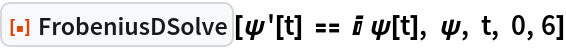Out=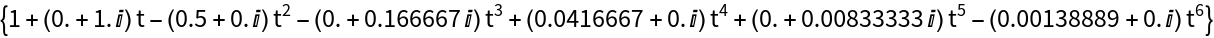Find a degree 10 approximate solution for the fourth-order ODE (x-1)4f''''[x]=x(2+x)f[x]+x2(x-1)2f''[x]-19(x-1)3f'''[x] near the singular point x=1:

 In:=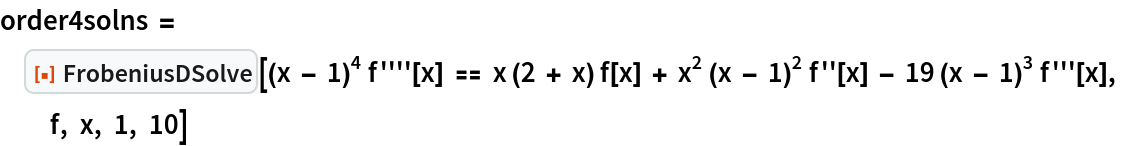Out=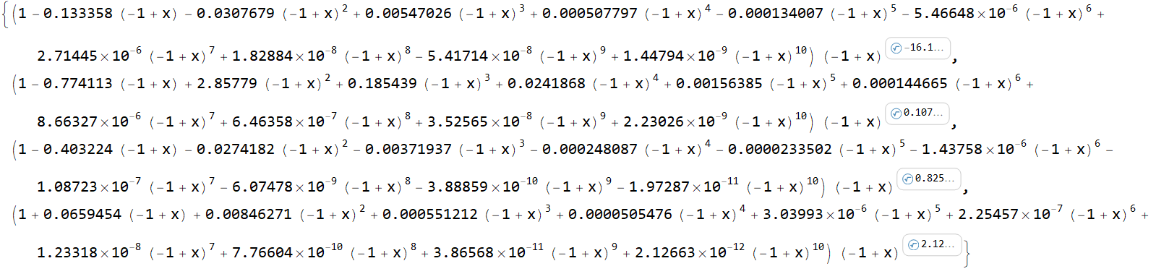Plot the results:

 In:=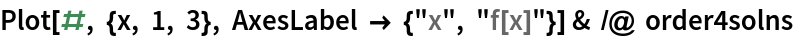Out=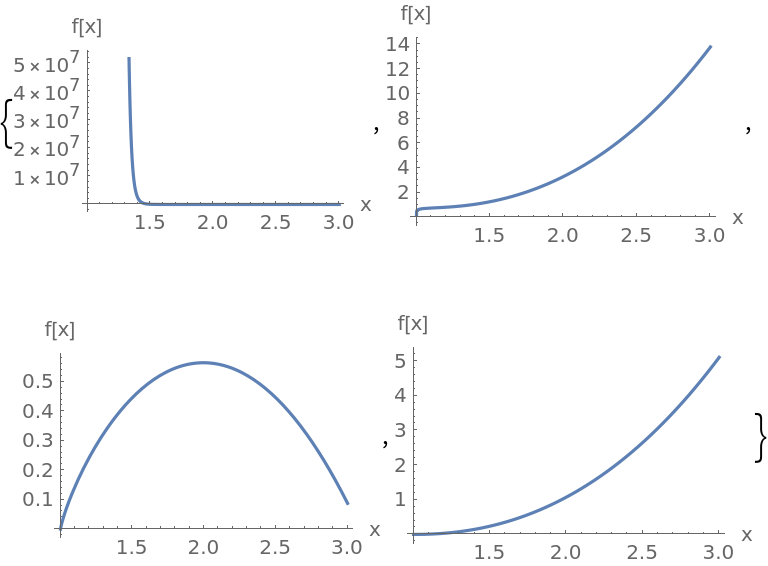The inputs of the function can be symbolics:

 In:=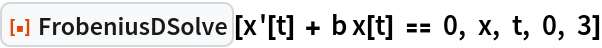Out=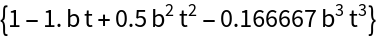In:=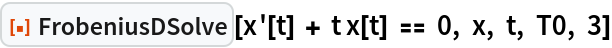Out=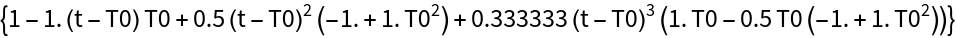### Options (1)

Set "ComputeError" to True to get a formula for the error, defined as the absolute value of the remainder, of a FrobeniusDSolve solution set (see Details and Options):

 In:=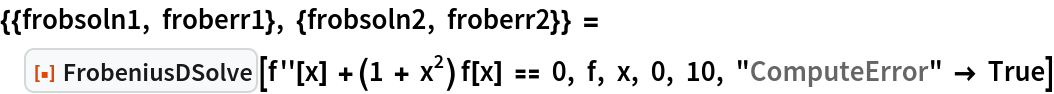Out=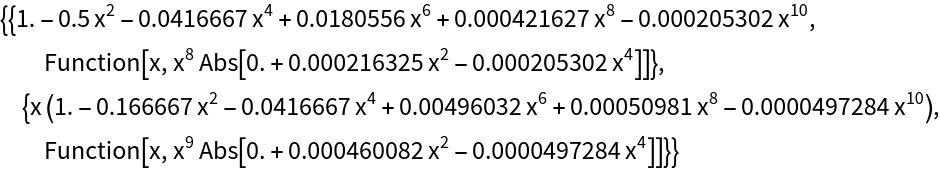### Properties and Relations (5)

Use AsymptoticDSolveValue to solve the ODE ty''[t]+y[t]=0 near the singular point t=0:

 In:=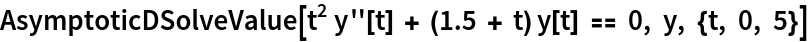Out=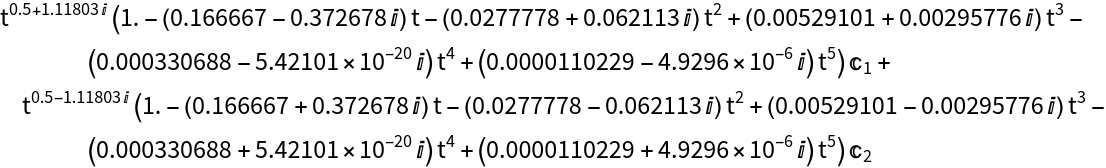Compare to the result from FrobeniusDSolve:

 In:=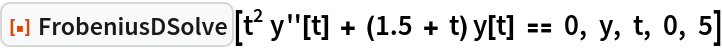Out=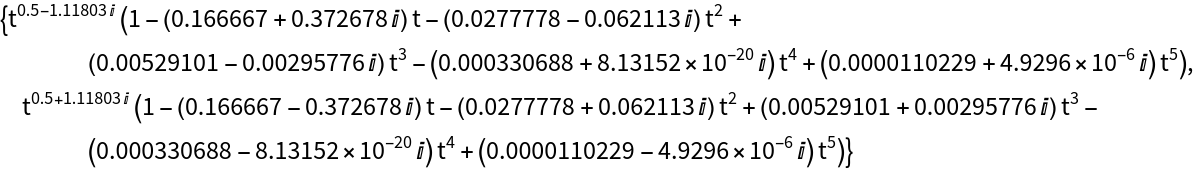Compare timings in milliseconds that FrobeniusDSolve and AsymptoticDSolveValue take to compute the 100th order power series approximation to y''+(1+x2)y=0:

 In:=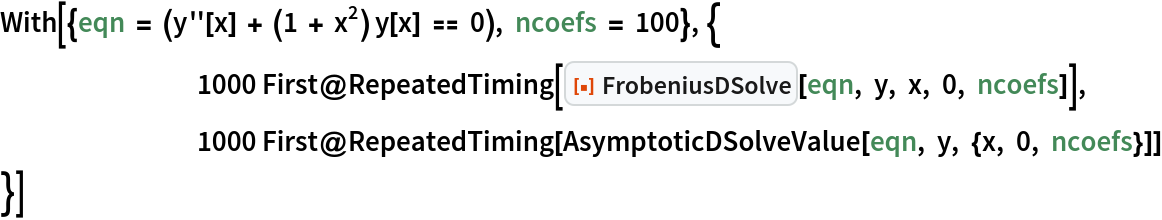Out=FrobeniusDSolve takes roughly 6 milliseconds to compute, while AsymptoticDSolveValue takes about 34. We can reduce computation time even further with the help of the resource function FrobeniusDSolveFormula:

 In:=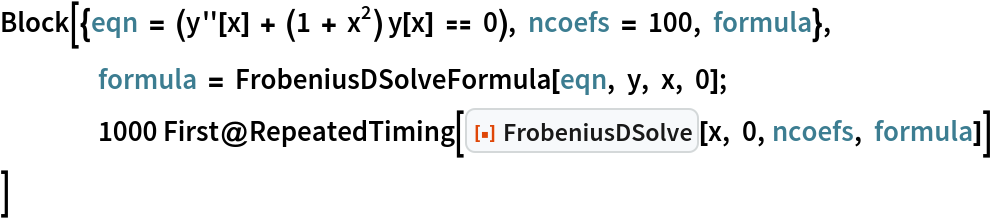Out=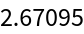When the option "ComputeError" is set to True and FrobeniusDSolveFormula is used, the option "ComputeError" should be switched to True in FrobeniusDSolveFormula, too:

 In:=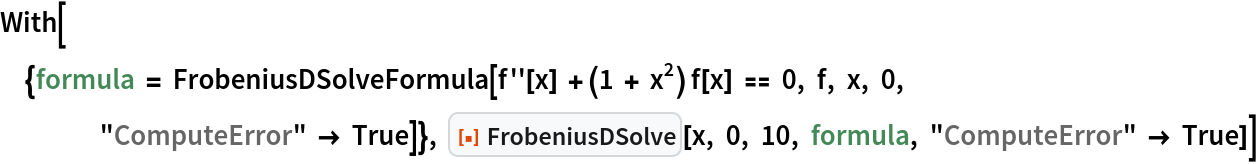Out=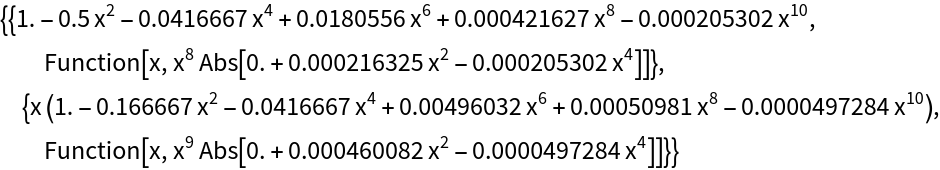### Possible Issues (3)

Solutions obtained using Frobenius's method generally only have a finite convergence radius. Try to compute an approximate solution to the ODE (1+x3)y'=y with n=60:

 In:=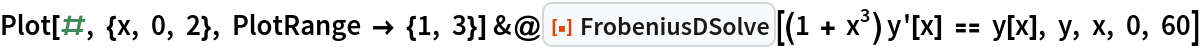Out=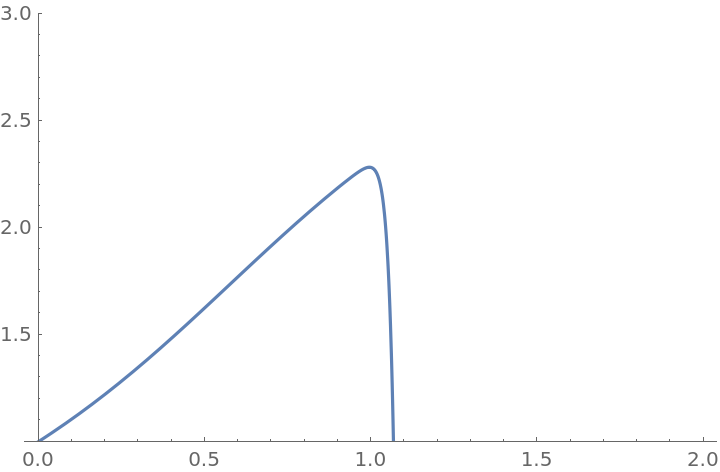The plot looks good for x<1, however, for x>1 the solution starts diverging. To see that, notice that the ODE (1+x3)y'=y demands y' to be positive for x>0, y>0; the plot, however, suddenly goes down at x=1. Besides, the sharpness of the "cliff" looks very suspicious.

Frobenius's method only works near ordinary points or regular singular points. If solution near an irregular singular point is attempted, Frobenius's method would only yield some of the possible solutions or won't be able to find any solutions at all. Consider the ODE x3y''+xy'+(x2-1)y=0 near the singular point x=0:

 In:=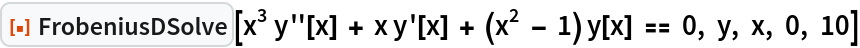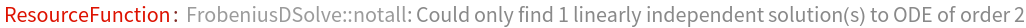Out=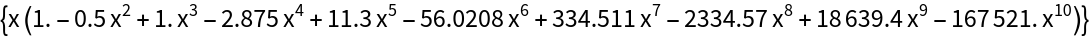FrobeniusDSolve could only give one of the two linearly independent solutions. The other solution could not be found because it cannot be written in the form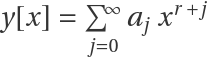, as can be seen with the help of AsymptoticDSolveValue:

 In:=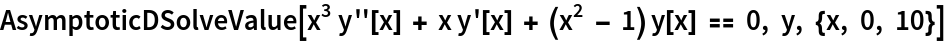Out=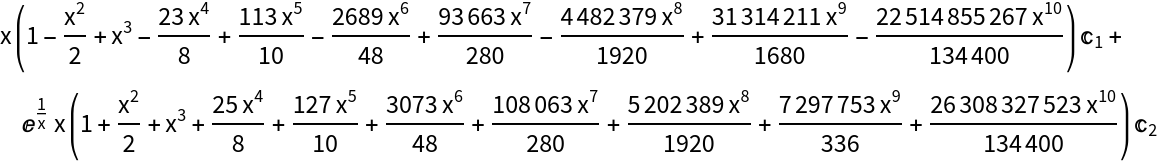The parameter n must be at least Z+M, where Z is the order of the ODE and M is the highest power of x (the independent variable). If the ODE is y''''[t]=-(1+t2)y[t], then the order Z=4 and the highest power of t is M=2, so n must be at least 6:

 In:=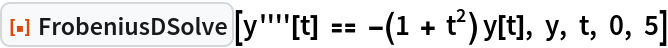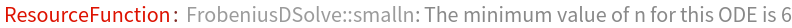Out=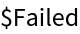### Neat Examples (2)

Sometimes, the Frobenius series solution to an ODE consists of a finite number of terms. For example, below are a few examples when the exact solution only has one term in it:

 In:=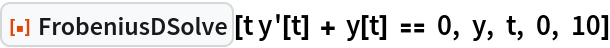Out=In:=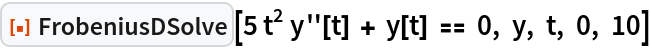Out=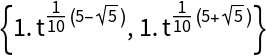Sometimes, the solution terminates after a couple terms. While the second of the two solutions below goes on forever, the first one only has four nonzero terms:

 In:=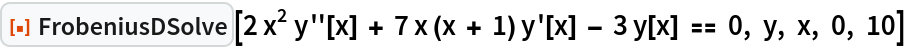Out=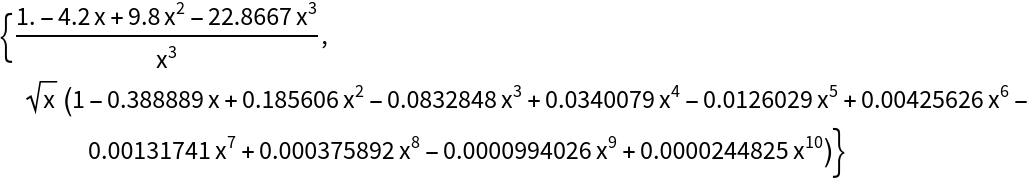In:=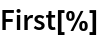Out=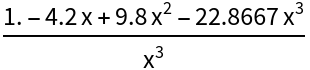## Publisher

Wolfram Summer Camp

## Version History

• 2.0.1 – 05 April 2021

## Author Notes

Plot the error of the approximate solutions to 2x2y''+9x(x+1)y'-3y=0 near the singular point x=0, with n=40 (obtained in Basic Examples):

 In:=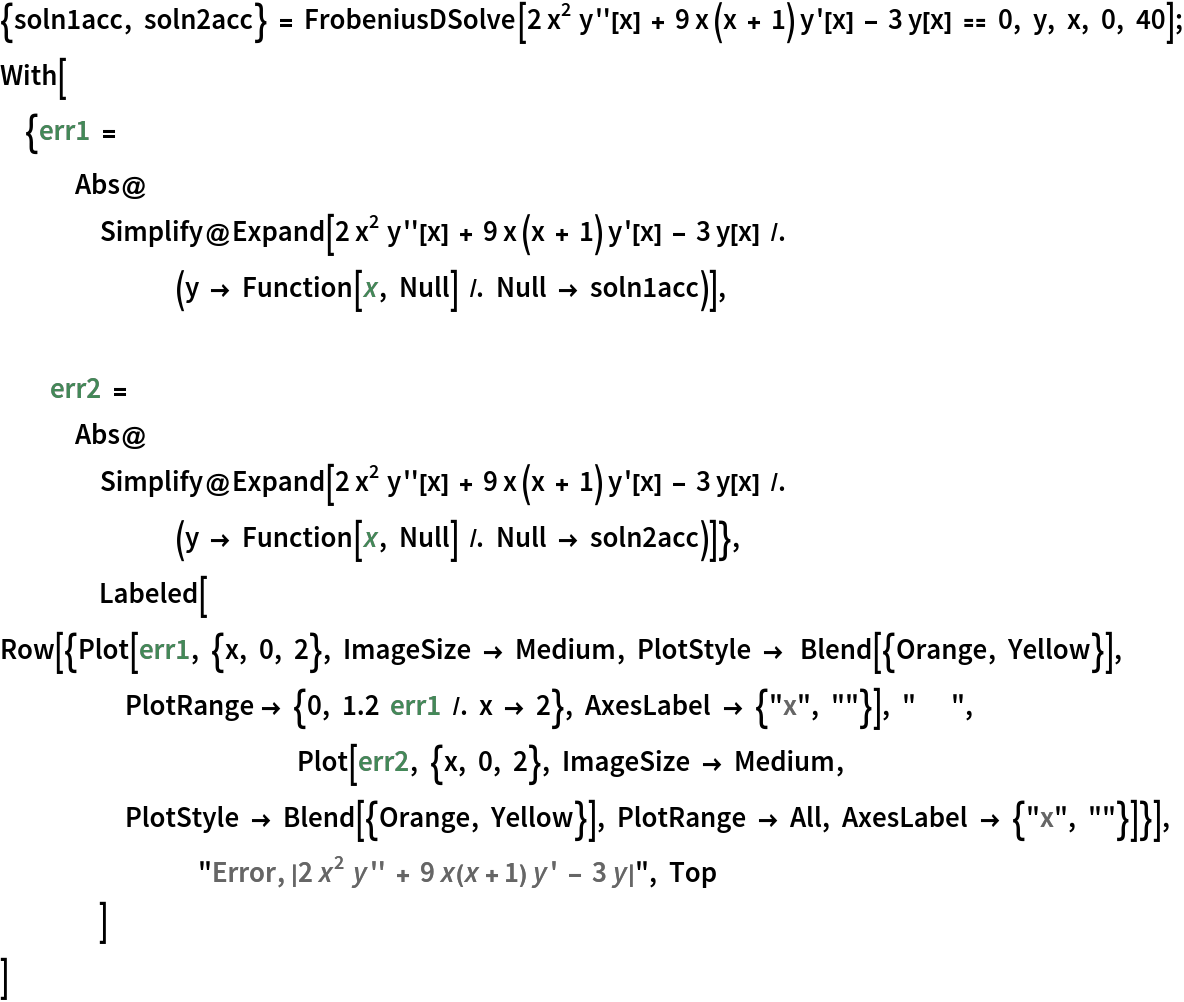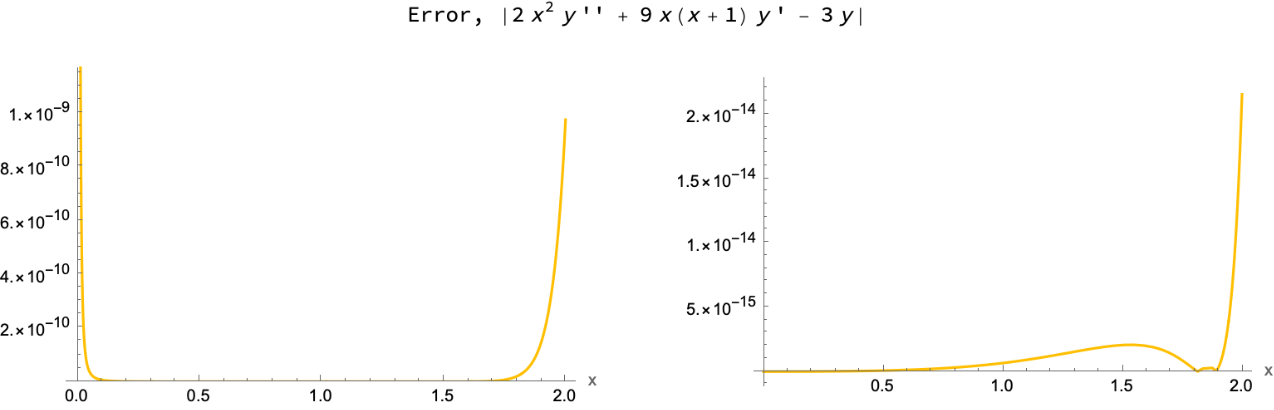The error of the first solution (the one proportional to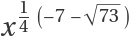) goes to infinity as x0 simply because there is some numerical error in the coefficients aj. When multiplied by, a tiniest error in aj leads to a huge residual, even though the true error is still very small. If, for example, the ODE to be solved is 0xy'+y=0, then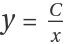; a small error in C leads to a huge residual at x0 but is insignificant for larger x. We can confirm the hypothesis by looking at the asymptotic expansion of the residual near x=0:

 In:=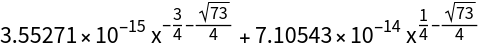The coefficients are of order 10-14, which is roughly the (default) numerical accuracy of the Wolfram Language.